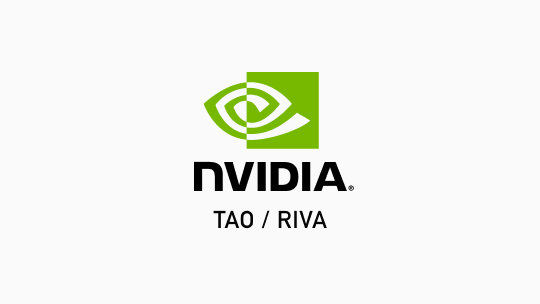NGC | Catalog
Welcome Guest#### Description

Question Answering Bert Base uncased model for extractive question answering on any provided content.

NVIDIA

#### Framework

Transfer Learning Toolkit

deployable_v1.0

April 8, 2022

418.15 MB

# Model Overview --------------

This is a checkpoint for Bert Base Uncased model for Question Answering trained on the question answering dataset SQuADv2.0 The model was trained for 2 epochs, with O1/16 bit mixed precision and batch size of 12 on 2 V100 GPUs. The model exact match (EM) score is 73.35% and an F1 score of 76.44%.

# Model Architecture ------------------

Apart from the BERT architecture this model also includes a question answering model head, which is stacked on top of BERT. This question answering head is a token classifier and is, more specifically, a single fully connected layer.

# Intended Use ------------

This model can be used as a part of the chat bot or semantic search system where it enables to find answers in the document to the factual questions To extend this for collections of the documents it is usually combined with Information retrieval systems on the first step, which return a small number of documents or passages that may contain the answer and will go as an input to the question answering model.

The model returns a concrete span of the answer in the content. If you want to create a conversational full sentence answer, you can either use some simple heuristics like, returning the full sentence where the answer is found. Or train another gnerative model, that can take the question and the answer span and generate a full sentence answer.

This QA model is trained on Squad dataset but it may also work on any new content without a need for retraining. But if you are working with specific technical language like for example medical one it may help first to fine tune the basic language model on this type of a content.

# Training Data -------------

The Question Answering model was trained on Squad2.0 dataset which combines the 100,000 questions in SQuAD1.1 with over 50,000 unanswerable questions written adversarially by crowdworkers to look similar to answerable ones. To do well on SQuAD2.0, systems must not only answer questions when possible, but also determine when no answer is supported by the paragraph and abstain from answering.

SQuAD 1.1, the previous version of the SQuAD dataset, contains 100,000+ question-answer pairs on 500+ articles from Wikipedia.

Training works as follows: The user provides training and evaluation data in text form in JSON format. This data is parsed by scripts in NeMo and converted into model input. The input sequence is a concatenation of a tokenized query and its according reading passage. The question answering head predicts for each token in the reading passage or context if it is the start or end of the answer span. The model is trained using cross entropy loss.

# Evaluation Metrics ------------------

With Extractive QA models, when the answer span is returned by the model, accuracy evaluation uses two metrics: The exact match (EM) and F1 score of the returned answer spans compared to the right answers. The overall EM and F1 scores are computed for a model by averaging the individual example scores.

• `Exact match`: If the answer span is exactly equal to the correct one, it returns 1; otherwise, it returns 0. When assessing against a negative example (SQuAD 2.0), if the model predicts any text at all, it automatically receives a 0 for that example.
• `F1`: The F1 score is a common metric for classification problems and widely used in QA. It is appropriate when we care equally about precision and recall. In this case, it's computed over the individual words in the prediction against those in the True Answer. The number of shared words between the prediction and the truth is the basis of the F1 score: Precision is the ratio of the number of shared words to the total number of words in the prediction, and recall is the ratio of the number of shared words to the total number of words in the ground truth. `F1 = 2 * (precision * recall) / (precision + recall)`

# How to Use This Model ---------------------

These model checkpoints are intended to be used with the Train Adapt Optimize (TAO) Toolkit. In order to use these checkpoints, there should be a specification file (.yaml) that specifies hyperparameters, datasets for training and evaluation, and any other information needed for the experiment. For more information on the experiment spec files for each use case, please refer to the TAO Toolkit User Guide.

Note: The model is encrypted and will only operate with the model load key `tao-encode`.

• To fine-tune from a model checkpoint (.tlt), use the following command (`` parameter should be a valid path to the file that specifies the fine-tuning hyperparameters, the dataset to fine-tune on, the dataset to evaluate on, epochs number):
``````!tao question_answering finetune -e \
-m \
-g
``````
• To evaluate an existing dataset using a model checkpoint (.tlt), use the following command (`` parameter should be a valid path to the file that specifies the dataset that is being evaluated):
``````!tao question_answering evaluate -e \
-m
``````